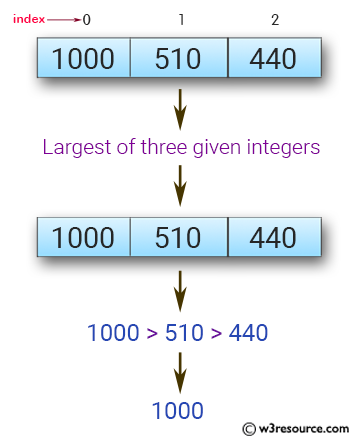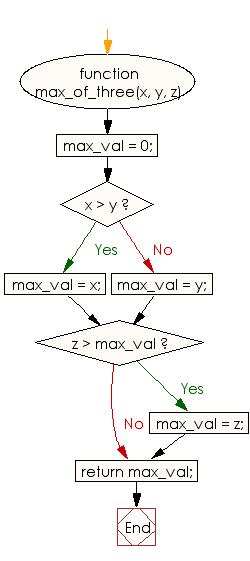# JavaScript: Find the largest of three given integers

## JavaScript Basic: Exercise-31 with Solution

Write a JavaScript program to find the largest of three given integers.

Pictorial Presentation:Sample Solution:

HTML Code:

``````<!DOCTYPE html>
<html>
<meta charset="utf-8">
<meta name="viewport" content="width=device-width">
<title>JavaScript program to find the largest of three given integers.</title>
<body>

</body>
</html>
```
```

JavaScript Code:

``````function max_of_three(x, y, z)
{
max_val = 0;
if (x > y)
{
max_val = x;
} else
{
max_val = y;
}
if (z > max_val)
{
max_val = z;
}
return max_val;
}

console.log(max_of_three(1,0,1));
console.log(max_of_three(0,-10,-20));
console.log(max_of_three(1000,510,440));
```
```

Sample Output:

```1
0
1000
```

Flowchart:ES6 Version:

``````function max_of_three(x, y, z)
{
max_val = 0;
if (x > y)
{
max_val = x;
} else
{
max_val = y;
}
if (z > max_val)
{
max_val = z;
}
return max_val;
}

console.log(max_of_three(1,0,1));
console.log(max_of_three(0,-10,-20));
console.log(max_of_three(1000,510,440));
``````

Live Demo:

See the Pen JavaScript: largest of three given integers - ex-31 by w3resource (@w3resource) on CodePen.

Improve this sample solution and post your code through Disqus

What is the difficulty level of this exercise?

Test your Programming skills with w3resource's quiz.

﻿

## JavaScript: Tips of the Day

Checks if a string is an anagram of another string (case-insensitive, ignores spaces, punctuation and special characters)

Example:

```const isAnagram = (str1, str2) => {
const normalize = str =>
str
.toLowerCase()
.replace(/[^a-z0-9]/gi, '')
.split('')
.sort()
.join('');
return normalize(str1) === normalize(str2);
};
console.log(isAnagram('iceman', 'cinema')); // true
```

Output:

```true
```